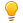We have been working very hard since 2009 to facilitate in your learning Read More. We can't keep up without your support. Donate Now.

#www.vustudents.ning.com

 www.bit.ly/vucodes + Link For Assignments, GDBs & Online Quizzes Solution www.bit.ly/papersvu + Link For Past Papers, Solved MCQs, Short Notes & More

//Created by Usman Khan.
//Basic idea from Pratyush Kumar

/**************************************************************************
Goldbach's Conjecture

Goldbach's conjecture is a rule in math that states the following: every even number greater than 2 can be expressed as the sum of two prime numbers.

Write a program that finds every possible pair of prime numbers, whose sum equals the given number or a set of numbers within a range.

For example:
Input: 16
Output:
3 + 13
5 + 11

Input: 32
Output:
3 + 29
13 + 19

Input: 4, 8
Output:
4: 2 + 2
6: 3 + 3
8: 3 + 5

***************************************************************************/

#include <iostream>
using namespace std;
#define ARRAY_SIZE 1000
int prime(int n)  // Function for checking prime numbers.
{
int c = 0;
for (int i = 2; i < n; i++)
{
if (n % i == 0)
{
c++;
}
}
if (c == 0)
{
return 1; //if not prime returns 1
}
else
{
return 0;
}
}

int main()
{
int rerun = 0;
do
{
int num;
int count = 0;
int a[ARRAY_SIZE];
int testarray1[ARRAY_SIZE], testarray2[ARRAY_SIZE];
int testnum1 = 0, testnum2 = 0;

cout "Enter any number less than 1000: " endl;
cin >> num; //getting numbers from user whose answer user wants.
for (int i = 2; i < num; i++) // from 2 to inputed number check every number
{
if (prime(i)) //if prime(i) returns 0 means number is prime it stores this number to the array.
{
a[count] = i;
count++; //count variable counts total number of prime numbers.
}
}
for (int j = 0; j <= count; j++)  //run loop on all prime numbers
{
for (int k = 0; k <= count; k++) //Again runs nested loop. To compare two numbers.  it looks like a two dimensional table or array.
{
if (a[k] + a[j] == num) //Checks if the one prime number (defined by outer for loop) + second prime number from the array a[] = the number entered by user.
{
for (int l = 0; l < ARRAY_SIZE; l++) //runs a loop for checking
{
if (testarray2[l] == a[k])  // checks if the one prime number is available in testarray2.
{
testnum1 = 1; // if available change testnum1 flag variable to 1.
}
}
for (int m = 0; m < ARRAY_SIZE; m++) //do the same for second prime number
{
if (testarray1[m] == a[j])
{
testnum2 = 1;
}
}

/******************************************
for (int l = 0; l < ARRAY_SIZE; l++) //runs a loop for checking
{
if (testarray2[l] == a[k])  // checks if the one prime number is available in testarray2.
{
testnum1 = 1; // if available change testnum1 flag variable to 1.
}
}
for (int m = 0; m < ARRAY_SIZE; m++) //do the same for second prime number
{
if (testarray1[m] == a[j])
{
testnum2 = 1;
}
}

This code checks and prevents the same numbers from printing again and again
on the screen.

For Example;
input: 10
10 = 3 + 7
10 = 5 + 5
10 = 7 + 3 //This prevents this type of repetations.
********************************************/

if ( !( testnum1 == 1 && testnum2 == 1) ) //Checks if the both falg variables are 1.
{

/********************
If both are one its means this combination is already printed.
so NOT operator makes condition false and skips this code.
Otherwise the combination is not yet printed and this codes executes.
*********************/

cout endl num " = " a[k] " + " a[j]; //prints anser.
testarray1[k] = a[k]; //saves first number in testarray1
testarray2[j] = a[j]; // saves second number in testarray2
}
}
}
}
cout endl "Do you want to check another number?" endl;
cout "Enter 1 to continue: " endl "Enter any other number to exit: " endl;
cin >> rerun;  // Gets input from user if user wnat to check another number or want to exit.
for (int n = 0; n < ARRAY_SIZE; n++)  //if user reuses programe we have to cleare old values and make code in free condition.
{
testarray1[n] = 0;
}
for (int p = 0; p < ARRAY_SIZE; p++) // these two loops make all values of testarray1 and 2 to zero.
{
testarray1[p] = 0;
}
} while (rerun == 1); // if answer of user is 1 condition become true and program run from start.
return 0;
}Tell me how's this and try yourself.

Run Program

+ http://bit.ly/vucodes (Link for Assignments, GDBs & Online Quizzes Solution)

+ http://bit.ly/papersvu (Link for Past Papers, Solved MCQs, Short Notes & More)